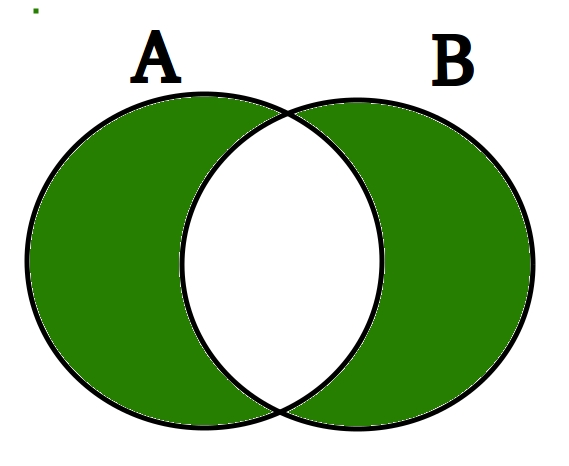# Python Set symmetric_difference()

The symmetric difference of two sets set1 and set2 is the set of elements which are in either of the sets set1 or set2 but not in both.Syntax :

`set1_name.symmetric_difference(set2_name) `

Parameters :
It only takes a single set as the parameter. If a list, tuple or dictionary is passed it converts it a set and performs the task.

Return value :

`Returns a set which is the symmetric difference between the two sets. `

Working Code for symmetric_difference() :

 `# Python program to demonstrate the use of  ` `# of symmetric_difference() method  ` ` `  ` `  `list1 ``=` `[``1``, ``2``, ``3``]  ` `list2 ``=` `[``2``, ``3``, ``4``]  ` `list3 ``=` `[``3``, ``4``, ``5``]  ` ` `  `# Convert list to sets ` `set1 ``=` `set``(list1)  ` `set2 ``=` `set``(list2)  ` ` `  `# Prints the symmetric difference when   ` `# set is passed as a parameter  ` `print``(set1.symmetric_difference(set2))  ` ` `  `# Prints the symmetric difference when list is  ` `# passed as a parameter by converting it to a set ` `print``(set2.symmetric_difference(list3)) `

Output :

```{1, 4}
{2, 5}
```
My Personal Notes arrow_drop_upCheck out this Author's contributed articles.

If you like GeeksforGeeks and would like to contribute, you can also write an article using contribute.geeksforgeeks.org or mail your article to contribute@geeksforgeeks.org. See your article appearing on the GeeksforGeeks main page and help other Geeks.

Please Improve this article if you find anything incorrect by clicking on the "Improve Article" button below.

Article Tags :
Practice Tags :

1

Please write to us at contribute@geeksforgeeks.org to report any issue with the above content.